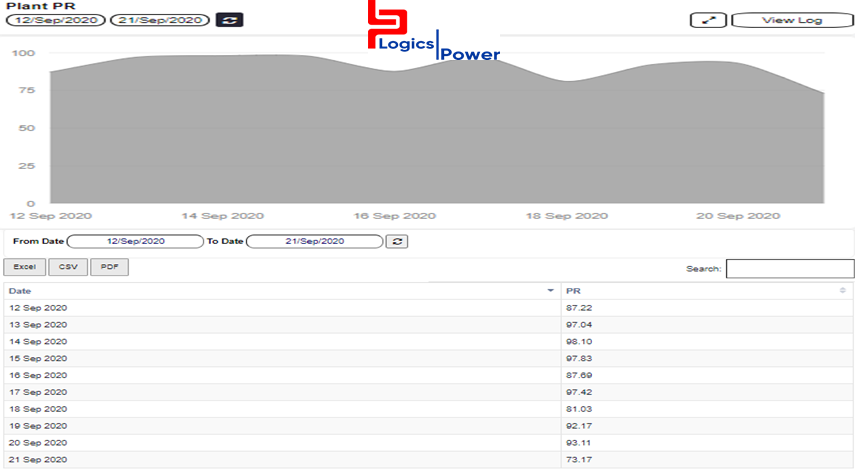# Blogs

Performance Ratio

#### Performance RatioPerformance Ratio is the most important parameter of a solar power plant. Specifically, the performance ratio is the ratio of the actual and theoretically possible energy outputs. It is largely independent of the orientation of a PV plant and the incident solar irradiation on the PV plant. It is the ratio of measured output to expected output for a given reporting period based on the system name-plate rating

Logics PowerAMR use the following formula for calculation of PR ratio- ((Energy generated / (installed plant capacity in KW *solar insolation in KWH/m2/ day))